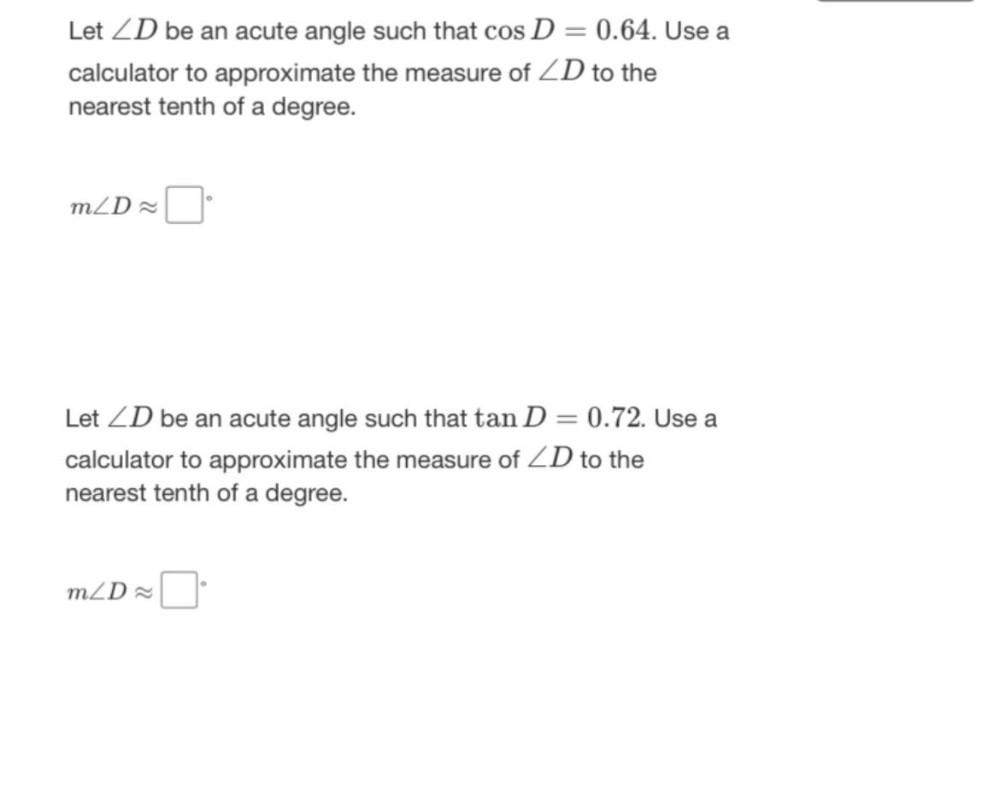Question:

# Let ∠D be an acute angle such that cos D=0.64. Use a calculator to approximate the measure of ∠D to the nearest tenth of a degree. m∠D ≈ _____. Let ∠D be an acute angle such that tan D = 0.72. Use a cLet ∠D be an acute angle such that cos D=0.64. Use a calculator to approximate the measure of ∠D to the nearest tenth of a degree. m∠D ≈ _____. Let ∠D be an acute angle such that tan D = 0.72. Use a calculator to approximate the measure of ∠D to the nearest tenth of a degree.